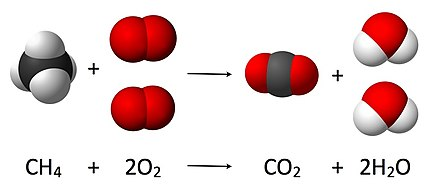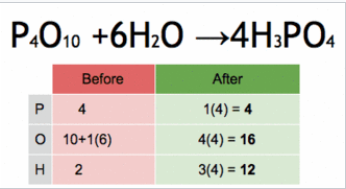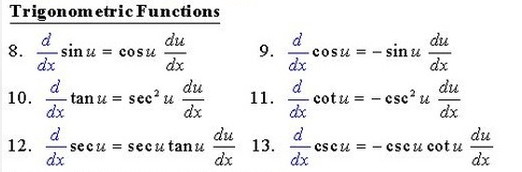# A conversion table for math and science

I have seen many ppl trying to recite numerous equations, theories, and notations. But everyone is in their own style, so a bit messy with ideas.

I propose a conversion table, for PAO users and other learners as a reference.Got an example of what it is we are trying to convert?

1 Like

I will upload it part by part. Please be patient.1 Like

Commonly used symbols up to high school level.

Persons imply variables/function_names.
Objects simply numbers.
Indicator technique for number string:
4563 = O45 O63 O2; the number string is even
38970 =O38 O97 O0 O1; the number string is odd
The last object will tell you the number string is even parity or odd parity. They need not be ‘2’ or ‘1’ in case many number string comes at the same time. Just randomly choose an odd number object for odd parity, or an even number object for even parity.

Startup any loci for scientific topics, for example, 4 loci with physic/chemistry/biology/math.

Rotting equations are not suggested, but if you have to, recite cleverly probably is the best approach in the worst scenario.

Example1:conversion: P3 P8 O4 A43 O2 P15 O2 A11 P3 P15 O2 A43 O2 P8 O2 P15
vsBalance the chemical equation by table.
from Chemical equation - Wikipedia

Example2:
To be continued, have to sleep!1 Like

Example2:
Differentiate trigonometric functionsAssign P30 = d/dx,
Assign P45 = sin,
Assign P29 = cos,
Assign P46 = tan,
Assign A18 = 1/x, reciprocal or inverse
such that
csc = 1/sin = P45 A18,
sec = 1/cos = P29 A18,
cot = 1/tan = P46 A18.

d/dx sin = cos, P30 P45 A61 P29,
d/dx sec = sec tan, P30 P29 A18 A61 P29 A18 P46

from

Once you learnt calculus, you will know the equations comes from taking the limit. d/dx the can simply treat as a slope.

When you did the exercise on d/dx trigonometry, you will find some interesting patterns.

1. d/dx the trigonometry with an initial c, eg cos, csc, cot output contain -ve.
2. d/dx with a character ‘t’, eg cot, tan output contain square.
3. d/dex sec or csc loves self-cloning.

And many more patterns are hidden in the equations.

The reason for memorizing the d/dx rules, when you are new to this math topic, you can have a great startup than others.

Even though you do not have experience with the topic, you can apply the rules to math formulas.

1 Like

Example3:

Instead following the formulas with given precedence.
Memorise them in an organised.
A killer’s story:
E = Pt,
P = IV, Look at that PEt! He is our VIP.
Q = It, Use electricity to kill (Q) it!

The flow chart I used from my physics exercise book, many years ago.

To conclude, it’s best if you can understand the formulas/rules/equations rationale behind them. If you can’t tell what’s the meaning of each variable, memorized the questions wouldn’t help you much. I believe ppl here are master of imagination, thus I think there is no need for more examples. Repeating my thesis, I intend to build a universal science symbol conversion table, which relieves the pain of mnemonic users, struggles of encoding the symbols into meaningful data.

2 Likes

Hello there,

Can you please exlain conversion of CH4 + 2O2 → CO2 + 2H2O again? I actually couldn’t understand it well.

For if you don’t have images for each element, you can use the conversion table. In the proposed table, C = Person 3, H = person 8, together CH means person 3 and 8 works together.
Else, you have an image for CH2 Methylene = a moth, you can use the moth to represent Ch2.

The conversion table suggests a brutal force of memorisation when you are in a hurry to recite.

Thank you.

1 Like

So, I get it, P3 means Person 3, A3 means action 3, O means Object 3?

So people represent alphabetic characters, actions represent operations, objects represent numbers?

Since there are a huge amount of possible symbols, it might be better to use actions unconnected to people to represent operations:

Also, it might be better grouping things by room - for example trigonometric derivatives. For example: have a room with a large image in the centre being the title “Trigonometric derivatives”, then just encode it going around the room by key-value pairs. Sin goes to cos, cos goes to negative sin etc.

In exams, etc. if you need to look up stuff, you just go straight to the room, rather than searching around. For example if you need to remember Table of Exact Values of Trigonometric Functions | Teaching Resources , then have a room for that with the images in rows and columns. That could speed things up.

1 Like